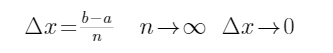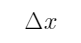The definite integral of a continues function like f(x) can be approximated by the sum of all narrow and tall rectangles areas obtained from partitioning  [a,b] interval into n equal sub intervals each with a length ofas shown in the figure. As n moves to a bigger number,the heights of each

rectangle would be a better approximation of the function values which results in a better area approximation, less error.

By approaching to infinity for n, the rectangles heights would be a perfect match to the whole function and results in the exact area bounded by the function and  x-axis from x=a to x=b.